﻿ 考虑回水效应的一库多级式水电站群短期优化调度模型 A Short-Term Optimal Scheduling Model of Cascaded Hydropower Stations with One Reservoir Considering the Effect of Backwater

Journal of Water Resources Research
Vol. 08  No. 03 ( 2019 ), Article ID: 30659 , 12 pages
10.12677/JWRR.2019.83030

A Short-Term Optimal Scheduling Model of Cascaded Hydropower Stations with One Reservoir Considering the Effect of Backwater

Han Gao, Jianjian Shen*, Chengguo Su, Chuntian Cheng, Xinyu Wu

Faculty of Infrastructure Engineering, Dalian University of Technology, Dalian LiaoningReceived: May 6th, 2019; accepted: May 24th, 2019; published: Jun. 5th, 2019ABSTRACT

The hydraulic coupling among cascaded hydropower stations with one reservoir is close, and the backwater from the downstream reservoir is easy to form a jacking effect on the tailwater level of the upstream reservoir, which affects the generation efficiency and planning. Based on the unit of the basic dispatching unit, this paper puts forward a short-term optimal scheduling model of cascaded hydropower stations with one reservoir, which takes the effect of backwater into consideration. Through the interval division of the downstream reservoir water level, the three-dimensional curve of tailwater level-discharge-reservoir water level is described as three typical scenarios, and the linear description is realized by piecewise linear method. The developed model converts into a mixed integer linear programming model, which can be solved by commercial software Lingo. Based on a case study with Tianshengqiao cascaded hydropower stations located in Hongshui River, the study shows that the proposed model considering backwater effect can realize the refined calculation and improve the economic benefits of scheduling.

Keywords:Cascaded Hydropower Stations with One Reservoir, Backwater, MILP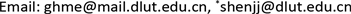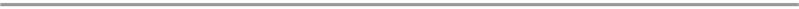“一库多级”梯级水电站间水力联系紧密，下库易产生回水对上库尾水位形成顶托作用，影响发电效率及计划安排。本文以机组为基本调度单元，提出了考虑回水效应的“一库多级”式水电站群短期优化调度模型，通过对下游库水位进行区间划分，将尾水位–泄量–库水位三维曲线描述为三种典型情境，采用分段线性方法实现了线性描述，最终将原问题转换为混合整数线性规划模型，并利用商用软件Lingo进行求解。经红水河干流天生桥梯级水电站群实例验证，提出的模型可在充分考虑回水影响下实现水电站群的精细化计算，有效提高梯级水电站群的发电量。1. 引言

2. 模型描述

2.1. 目标函数

$E=\mathrm{max}\underset{i=1}{\overset{I}{\sum }}\underset{n=1}{\overset{Ni}{\sum }}\underset{t=1}{\overset{T}{\sum }}{p}_{i,n,t}\Delta t$ (1)

2.2.约束条件

1、线性约束

1) 水量平衡约束

${V}_{i,t}={V}_{i,t-1}+3600\left({I}_{i,t}+I{F}_{i,t}-{Q}_{i,t}\right)×\Delta t$ (2)

${Q}_{i,t}=\underset{n=1}{\overset{{N}_{i}}{\sum }}{q}_{i,n,t}+{S}_{i,t}$ (3)

2) 始、末库容限制

${V}_{i,1}={V}_{i}^{Initial}$ (4)

${V}_{i,T}={V}_{i}^{End}$ (5)

3) 水库边界条件

$\underset{_}{{V}_{i}}\le {V}_{i,t}\le \stackrel{¯}{{V}_{i}}$ (6)

$\underset{_}{{Q}_{i}}\le {Q}_{i,t}\le \stackrel{¯}{{Q}_{i}}$ (7)

$0\le {S}_{i,t}\le \stackrel{¯}{{S}_{i,t}}$ (8)

4) 机组出力限制

$\left\{\begin{array}{l}{w}_{i,n,t}\underset{_}{{p}_{i,n}}\le {p}_{i,n,t}\le {w}_{i,n,t}\stackrel{¯}{{p}_{i,n}}\\ {w}_{i,n,t}\in \left\{0,1\right\}\end{array}$ (9)

5) 机组发电流量限制

${w}_{i,n,t}\underset{_}{{q}_{i,n}}\le {q}_{i,n,t}\le {w}_{i,n,t}\stackrel{¯}{{q}_{i,n}}$ (10)

6) 机组开、停机状态约束

${x}_{i,n,t}-{\stackrel{˜}{x}}_{i,n,t}={w}_{i,n,t}-{w}_{i,n,t-1}$ (11)

${x}_{i,n,t}+{\stackrel{˜}{x}}_{i,n,t}\le 1$ (12)

${x}_{i,n,t},{\stackrel{˜}{x}}_{i,n,t}\in \left\{0,1\right\}$ (13)

7) 机组开、停机持续时间约束及最大开机次数约束

${x}_{i,n,t}+\underset{\lambda =t+1}{\overset{t+{T}_{i,n}^{On}-1}{\sum }}{\stackrel{˜}{x}}_{i,n,\lambda }\le 1$ (14)

${\stackrel{˜}{x}}_{i,n,t}+\underset{\lambda =t+1}{\overset{t+{T}_{i,n}^{Off}-1}{\sum }}{x}_{i,n,\lambda }\le 1$ (15)

$\underset{t=1}{\overset{T}{\sum }}{x}_{i,n,t}\le {X}_{i,n}^{Max}$ (16)

8) 机组发电水头约束

${h}_{i,n,t}=\frac{{Z}_{i,t-1}+{Z}_{i,t}}{2}-{Z}_{i,t}^{d}-{h}_{i,n,t}^{loss}$ (17)

2、非线性约束

1) 水位库容约束

${Z}_{i,t}={f}_{i,zv}\left({V}_{i,t}\right)$ (18)

2) 尾水位泄量约束

${Z}_{i,t}^{d}={f}_{i,zqz}\left({Q}_{i,t},{Z}_{i+1,t}\right)$ (19)

3) 机组动力特性约束

${p}_{i,n,t}={f}_{i,n,pqh}\left({q}_{i,n,t},{h}_{i,n,t}\right)$ (20)

4) 机组振动区限制

$\left({p}_{i,n,t}-\stackrel{¯}{{p}_{i,n}^{k}}\right)\left({p}_{i,n,t}-\underset{_}{{p}_{i,n}^{k}}\right)\ge 0$ (21)

3. 求解算法

3.1. 水位库容约束处理

$\left\{\begin{array}{l}{Z}_{i}^{a}={f}_{i,zv}\left({V}_{i}^{a}\right)\\ \underset{_}{{V}_{i}}={V}_{i}^{0}<{V}_{i}^{1}<\cdots <{V}_{i}^{a}<\cdots <{V}_{i}^{A}=\stackrel{¯}{{V}_{i}}\end{array}$ (22)

$\underset{a=1}{\overset{A}{\sum }}{r}_{i,t}^{a}=1$ (23)

${r}_{i,t}^{a}\in \left\{0,1\right\}$ (24)

${r}_{i,t}^{a}{V}_{i}^{a-1}\le {V}_{i,t}^{a}\le {r}_{i,t}^{a}{V}_{i}^{a}$ (25)

$\underset{a=1}{\overset{A}{\sum }}{V}_{i,t}^{a}={V}_{i}^{a}$ (26)

${Z}_{i,t}=\underset{a=1}{\overset{A}{\sum }}\left[{r}_{i,t}^{a}{Z}_{i}^{a-1}+\frac{{Z}_{i}^{a}-{Z}_{i}^{a-1}}{{V}_{i}^{a}-{V}_{i}^{a-1}}\cdot \left({V}_{i,t}^{a}-{r}_{i,t}^{a}{V}_{i}^{a-1}\right)\right]$ (27)

3.2. 尾水位泄量约束处理Figure 1. Three-dimensional curves of tail water level - discharge - reservoir water level

3.2.1. 下游水库库水位离散

$\left\{\begin{array}{l}\underset{k=1}{\overset{3}{\sum }}{\beta }_{i+1,t}^{k}=1\\ {\beta }_{i+1,t}^{k}\in \left\{0,1\right\}\\ \underset{k=1}{\overset{3}{\sum }}{\beta }_{i+1,t}^{k}{Z}_{i+1}^{k-1}\le {Z}_{i+1,t}^{k}\le \underset{k=1}{\overset{3}{\sum }}{\beta }_{i+1,t}^{k}{Z}_{i+1}^{k}\end{array}$ (28)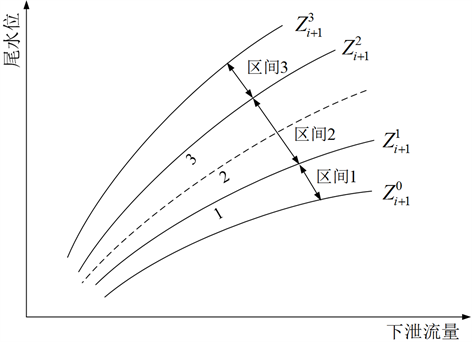Figure 2. Simplified curves of tail water level-discharge-reservoir water level

3.2.2. 下游水库坝前水位关联尾水位泄量曲线

$-\stackrel{¯}{{Z}_{i}^{d}}\left(1-{\beta }_{i+1,t}^{1}\right)\le {Z}_{i,t}^{d}-{f}_{i,zq|z}^{1}\left({Q}_{i,t}\right)\le \stackrel{¯}{{Z}_{i}^{d}}\left(1-{\beta }_{i+1,t}^{1}\right)$ (29)

$-\stackrel{¯}{{Z}_{i}^{d}}\left(1-{\beta }_{i+1,t}^{2}\right)\le {Z}_{i,t}^{d}-{f}_{i,zq|z}^{2}\left({Q}_{i,t}\right)\le \stackrel{¯}{{Z}_{i}^{d}}\left(1-{\beta }_{i+1,t}^{2}\right)$ (30)

$-\stackrel{¯}{{Z}_{i}^{d}}\left(1-{\beta }_{i+1,t}^{3}\right)\le {Z}_{i,t}^{d}-{f}_{i,zq|z}^{3}\left({Q}_{i,t}\right)\le \stackrel{¯}{{Z}_{i}^{d}}\left(1-{\beta }_{i+1,t}^{3}\right)$ (31)

3.2.3. 尾水位泄量曲线线性化转换

3.2.2节中被选定的尾水位泄量二维曲线仍然为非线性曲线，不可直接运用到MILP模型中参与计算。参考3.1节中水位库容约束的处理方法，离散下泄流量，线性插值得到相应的尾水位，实现尾水位泄量曲线的线性处理。详细步骤此处不再赘述。

3.3. 机组动力特性约束处理

3.4. 机组振动区限制处理

$\left\{\begin{array}{l}\underset{k=1}{\overset{K+1}{\sum }}{\alpha }_{i,n,t}^{k}={u}_{i,n,t}\\ {\alpha }_{i,n,t}^{k}\in \left\{0,1\right\}\\ \underset{k=1}{\overset{K+1}{\sum }}{\alpha }_{i,n,t}^{k}\underset{_}{{p}_{i,n,f}^{k}}\le {p}_{i,n,t}\le \underset{k=1}{\overset{K+1}{\sum }}{\alpha }_{i,n,t}^{k}\stackrel{¯}{{p}_{i,n,f}^{k}}\end{array}$ (32)

4. 应用实例

4.1. 工程背景Table 1. Characteristic parameters of cascaded hydropower plants

4.2. 计算结果及分析

4.2.1 . 汛期计算结果分析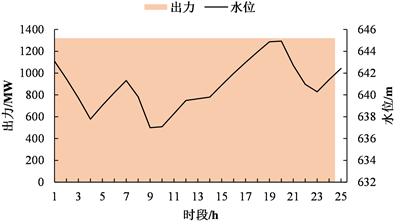(a) 天生桥一级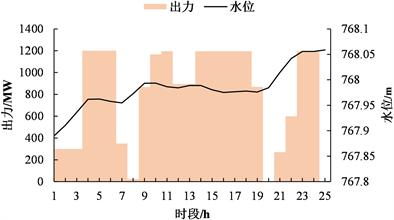(b) 天生桥二级

Figure 3. Power output and water level of hydropower plants in flood season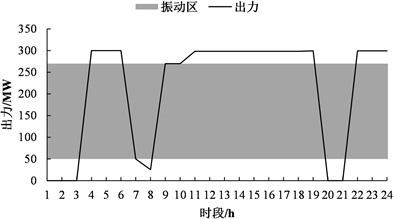(a) 天生桥一级1#机组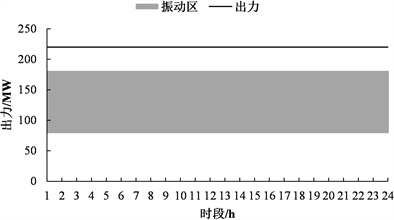(b) 天生桥二级1#机组

Figure 4. Power output of units in flood season

4.2.2. 枯期计算结果分析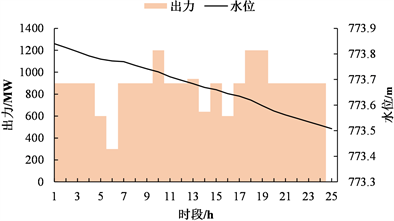(a) 天生桥一级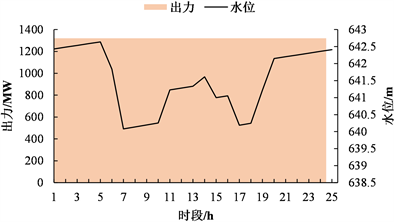(b) 天生桥二级

Figure 5. Power output and water level of hydropower plants in dry season

4.2.3. 考虑回水与未考虑回水模型尾水位对比分析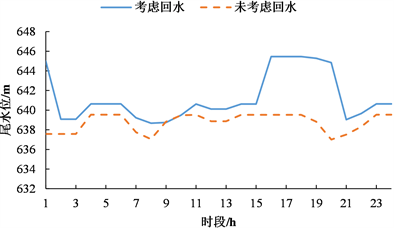(a) 汛期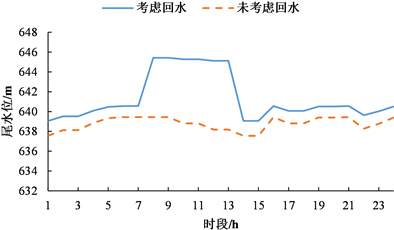(b) 枯期

Figure 6. Comparison of tail water level with and without considering backwater

5. 结语

1) 本文构建了“一库多级”水电站群短期调度MILP模型，重点处理了梯级水头联系：尾水位–泄量–库水位三维曲线。

2) 针对模型中的水位库容关系、尾水位–泄量–库水位关系、机组动力特性曲线以及机组振动区等非线性约束，采用分段线性化方法拟合，保留了原约束的特性，较好地贴近实际工况。

3) 以机组为基本调度单元，能有效满足振动区、机组开停机持续时间及最大开机次数约束等约束，保证了机组的稳定运行。

4) 采用Lingo软件求解红水河梯级实例，尽管模型中的约束和整数变量数目庞大，仍可在可接受的时间范围内求得全局最优解，为涉及回水问题的“一库多级”水电站群短期调度提供一种可靠的解决方法。

A Short-Term Optimal Scheduling Model of Cascaded Hydropower Stations with One Reservoir Considering the Effect of Backwater[J]. 水资源研究, 2019, 08(03): 248-259. https://doi.org/10.12677/JWRR.2019.83030

1. 1. CHENG, C., YAN, L., MIRCHI, A., et al. China’s booming hydropower: Systems modeling challenges and opportunities. Journal of Water Resources Planning and Management, 2016, 143(1): 02516002. https://doi.org/10.1061/(ASCE)WR.1943-5452.0000723

2. 2. 申建建, 张楠男, 程春田, 等. 基于聚类分析和决策树的“一库多级”水电站日调度方法[J]. 中国电机工程学报, 2019, 39(3): 652-663+945. SHEN Jianjian, ZHANG Nannan, CHENG Chuntian, et al. Method for daily operation of hydropower stations with one reservoir based on cluster analysis and decision tree technique. Proceedings of the CSEE, 2019, 39(3): 652-663+945. (in Chinese)

3. 3. WANG, J. Short-term generation scheduling model of Fujian hydro system. Energy Conversion and Management, 2009, 50(4): 1085-1094. https://doi.org/10.1016/j.enconman.2008.12.004

4. 4. 申建建, 程春田, 李卫东, 等. 复杂时段耦合型约束水电站群短期变尺度优化调度方法[J]. 中国电机工程学报, 2014, 34(1): 87-95. SHEN Jianjian, CHENG Chuntian, LI Weidong, et al. A mutative-scale optimization method for short-term operation of hydropower plants with complex temporal coupling constraints. Proceedings of the CSEE, 2014, 34(1): 87-95. (in Chinese)

5. 5. 李义, 李承军, 周建中. POA-DPSA混合算法在短期优化调度中的应用[J]. 水电能源科学, 2004, 22(1): 37-39. LI Yi, LI Chengjun and ZHOU Jianzhong. POA-DPSA algorithm for short-term hydro scheduling of cascaded hydroelectric stations. Water Resources and Power, 2004, 22(1): 37-39. (in Chinese)

6. 6. JI, C., YU, H., WU, J., et al. Research on cascade reservoirs’ short-term optimal operation under the effect of reverse regulation. Water, 2018, 10(6): 808. https://doi.org/10.3390/w10060808

7. 7. 苏承国, 王沛霖, 武新宇, 等. 考虑机组组合的梯级水电站短期调峰MILP模型[J]. 电网技术, 2018, 42(6): 1883-1891. SU Chengguo, WANG Peilin, WU Xinyu, et al. A compact MILP model for short-term peak shaving of cascaded hydropower plants considering unit commitment. Power System Technology, 2018, 42(6): 1883-1891. (in Chinese)

8. 8. 徐鼎甲. 进行日调节时水电站下游水位的计算[J]. 水利水电技术, 1995(4): 2-4. XU Dingjia. Calculation of downstream water level of hydropower station during daily regulation. Water Resources and Hydropower Engineering, 1995(4): 2-4. (in Chinese)

9. 9. LIU, B., CHENG, C., WANG, S., et al. Parallel chance-constrained dynamic programming for cascade hydropower system operation. Energy, 2018, 165: 752-767. https://doi.org/10.1016/j.energy.2018.09.140

10. 10. 纪昌明, 马皓宇, 李传刚, 等. 基于可行域搜索映射的并行动态规划[J]. 水利学报, 2018, 49(6): 649-661. JI Changming, MA Haoyu, LI Chuangang, et al. Research on parallel dynamic programming based on feasible region search mapping. Journal of Hydraulic Engineering, 2018, 49(6): 649-661. (in Chinese)

11. 11. 梅亚东. 梯级水库优化调度的有后效性动态规划模型及应用[J]. 水科学进展, 2000, 11(2): 194-198. MEI Yadong. Dynamic programming model without markovin property of cascade reservoirs operation and its application. Advances in Water Science, 2000, 11(2): 194-198. (in Chinese)

12. 12. CATALAO, J. P. S., MARIANO, S. J. P. S., MENDES, V. M. F., et al. Scheduling of head-sensitive cascaded hydro systems: A nonlinear approach. IEEE Transactions on Power Systems, 2009, 24(1): 337-346. https://doi.org/10.1109/TPWRS.2008.2005708

13. 13. BORGHETTI, A., et al. An MILP approach for short-term hydro scheduling and unit commitment with head-dependent reservoir. IEEE Transactions on Power Systems, 2008, 23(3): 1115-1124. https://doi.org/10.1109/TPWRS.2008.926704

14. 14. CHENG, C., WANG, J. and WU, X. Hydro unit commitment with a head-sensitive reservoir and multiple vibration zones using MILP. IEEE Transactions on Power Systems, 2016, 31: 4842-4852. https://doi.org/10.1109/TPWRS.2016.2522469

15. 15. GE, X. L., ZHANG, L. Z., SHU, J., et al. Short-term hydropower optimal scheduling considering the optimization of water time delay. Electric Power Systems Research, 2014, 110: 188-197. https://doi.org/10.1016/j.epsr.2014.01.015